A Gas Laws Primer

by

Larry "Harris" Taylor, Ph.D

This is an electronic reprint and expansion of an article that appeared in Sources (July/August, 1992, 49-52.) This material is copyrighted and all rights retained by the author. This article is made available as a service to the diving community by the author and may be distributed for any non-commercial or Not-For-Profit use.

Fini

Jump To: Kinetic     Absolute Values     Charles     Guy-Lussac     Boyle     General     Dalton     Henry     Real     VanDer Waal

KINETIC THEORY OF GASES

The behavior of gases in the physical world is a major influence on the diver. Since the changes in gas volume related to pressure and temperature effects buoyancy, as well as the amount of breathing gas available to the diver, understanding some basic principles of gas characteristics can dramatically increase diver comfort and safety.

All gases, irrespective of chemical composition, demonstrate similar behavior in response to physical changes of composition, temperature, and pressure. This uniformity of physical behavior was of great interest to early scientists. It is one of the dogmas of science that the behavior of a material is a reflection of the particles that make up the substance. In other words, the differences between a solid, liquid, and gas reflect the movement of the small particles (atoms) that compose all matter. This assumption is part of the Kinetic Theory Of Gases (Kinetic is from the Greek word for motion).   Historically, the description of gas properties has been summarized by the mathematical equations describing the relationships between gas volume, pressure and temperature. These equations, named after the scientist who first demonstrated their validity, have been labeled the "gas laws." These gas laws are best understood within the framework described as the kinetic theory of gases:

1. Gases are composed of molecules.

2. These molecules are in constant motion. This is why gases mix to uniformity and fill all portions of the containment vessel.

3. Molecules of a gas collide frequently with each other and with the walls of the containment vessel.

4. Under ordinary circumstances, the distance between gas molecules is far greater than the size of the individual molecules. This is why gases can be compressed.

5. The molecules of a gas move in all directions with an average velocity at a given temperature. At a given temperature, the average energy of molecules in the gaseous state is the same for all substances.

6. Molecules are perfectly elastic; thus they lose no energy when they collide with another molecule. (If they lost energy on collision, the temperature of a gas mix would always decrease with time.)

These six assumptions are the basis for understanding gas behavior. For example, the measurement of the "intensity" (force per unit area) of the collisions of the gas molecules with the walls of the containment vessel is termed pressure. As we increase the kinetic energy (by raising temperature) the molecules will have more velocity and will collide with more force more often on the vessel walls. This is why pressure increases in a closed container as temperature is raised.

One way of conceptualizing this is to think of gas molecules like little metal pellets (bb's). As they move about in containment, they will strike the walls of the container. In the illustration below, these gas "pellets" are randomly moving inside a cylinder. At the right end of the cylinder in the illustration is a piston, held in place by some spring mechanism within the brown box.  As the gas molecules strike the flat plate of the piston, the combined force of all the impacts will move the piston backwards until the force of the gas molecules (bb's)  striking the flat plate of the piston balances a spring device contained within the brown box. A measurement of this impact is displayed on a mechanical gauge. If we increase the temperature, we increase the average velocity of the gas molecules. This means they will strike the flat plate faster (with more force) and the plate will move within the cylinder  to indicate a higher pressure.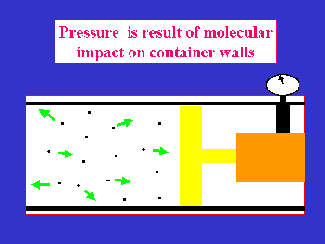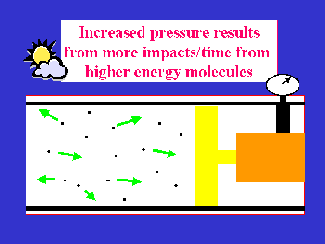GAS LAW FUNDAMENTALS

The relationship between temperature, pressure, and volume has been extensively studied. Historically, the behavior of gases was evaluated by measuring the temperature, pressure and volume of the material under study. Because of the complexity of attempting to simultaneously measure and predict all values, typically, one of the values (P   (Pressure) , V  (Volume) or T (Temperature) ) was held constant. Then another value was changed to determine that change on the third parameter.. For example, an experimenter would hold pressure constant (stay at same altitude above sea level) and measure the gas volume change (in a flexible container) as the temperature was varied. In turn, over time, all of the possible combinations were examined and each situation has been given a name for the scientist who firmly established the validity of the particular relationship that is now called a gas law. Association of scientist's name with the mathematical relationship describing gas behavior under some set of specified conditions is one way of remembering these gas laws.

ABSOLUTE VALUES

The magnitude or behavior of gases is best illustrated by looking at some numerical examples. It is important to remember that in all gas law problems,  the relationships are valid only when ABSOLUTE UNITS for pressure and temperature are used. In other words, to make the equation describe reality, absolute values must be used. Absolute temperature was originally defined when the linear relationship between temperature and volume was discovered (See Charles Law, below). So, not using absolute temperature most likely means you are not in a range where the relationship between temperature and volume is linear and any solution will be incorrect.

Since we live under a gaseous  blanket (and this gas has weight and thus exerts a force called pressure), the weight of this volume must be considered because gases behave according to the TOTAL pressure acting on them. We typically label our pressure gauges 0 because for many applications (measuring pressure within a container) we are NOT CONCERNED with the behavior of gases; we simply want to know if the pressure measure on the gauge is less than the tolerated pressure (to prevent an explosion) of the container or, as in scuba diving, we use the pressure gauge as a measure of remaining gas (see Estimating Gas Consumption).. However, when we choose to examine or predict the behavior of gases, we MUST consider all the forces involved. So, absolute pressure is defined as the pressure we measure (on the gauge) plus the pressure exerted from the weight of the atmosphere. This takes the form of this equation:

P= P +   P atm   where

 Pa  = Absolute pressure Pg = Gauge pressure Patm = Atmospheric pressure

All of these measurements must be in the same units. Typically, in diving, we use atmospheres as a measure of pressure  because this makes the pressure of the atmosphere defined as 1.0. Thus, when using atmospheres, the above equation becomes

P= P +   1.0

It is very important that measurements be in the same units. Mixing units (gauge pressure in psi or bar and atmospheric pressure as 1) will lead to the wrong numerical value for your solution. One  of the most common sources of error is NOT USING absolute pressure and temperature values. So, get into the habit of converting to absolute units whenever you enter the realm of solving gas related problems. Finally, when the weight of the atmosphere changes (as in going to altitude where there is less overhead weight of the atmosphere) the numerical value for  P atm will change (See Altitude Arithmetic ).

NOTE: It makes no difference if the units of pressure are expressed in psi, mm Hg, cm H2O, bars, kilopascals, g/sq cm, kg/sq m, etc. However, it is necessary to use absolute measurements and to keep the units the same in both starting and ending conditions being considered.

CHARLES' LAW

The French scientist, Jacques Charles, in 1787, studied the relationship between temperature and volume at constant pressure. This study was prompted by an attempt to understand how hot air balloons worked. He noted that in the vicinity of zero degrees Celsius, the volume of a gas decreased by a factor of 1/273 for each degree Celsius decrease. If one theoretically continued this decrease in temperature, then a gas would have zero volume at -273 degrees C. This value is termed Absolute Zero. (If a gas has zero volume, then there will be no molecular motion (velocity = zero in the kinetic energy equation) and thus no kinetic energy). Measurements of temperature based on this absolute zero point are termed Absolute Temperature. (Since gases will liquefy before absolute zero is reached, the "zero volume" state is not obtainable.) Since this 1/273 change in volume corresponds to one degree change on the absolute temperature scale, absolute temperatures are used when using "the gas laws" described below to predict variations in pressure, temperature, and volume. Charles' observations have been formalized into Charles' Law:

The volume of a gas at constant pressure is directly proportional to the absolute temperature.

A practical example of Charles law involves the effect on the volume (size) of any flexible container with change in temperature. Inner tunes, surface floats, etc. will increase in volume when heated and decrease in volume when chilled. So  will the volume of gas in a buoyancy compensator when passing through thermoclines. So, the effect of buoyancy from slight changes in pressure when moving through water column zones with dramatically different temperatures will be exacerbated by this temperature effect on volume. Thus, as the gas chills after entering a thermocline, buoyancy will continue to decrease until the temperature of the gas in the bc is the same temperature as the ambient water. Likewise, on ascent out of the thermocline, the movement into warmer water  will cause an increase in buoyancy from the expansion of the gas in the bc related to this temperature effect.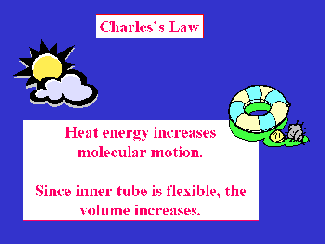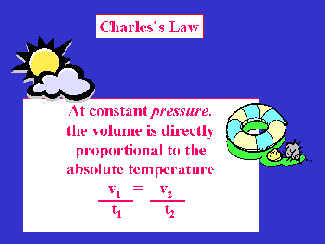ENGLISH EXAMPLE: If a scuba cylinder is capable of delivering 40 ft3 of air to a diver at 78 oF, how much air is available at 55 oF?

First, determine absolute temperature (T) at the two conditions (1 and 2) being considered:

T1 = 78 oF + 460 = 538 oR

T2 = 55 oF + 460 = 515 oR

NOTE: The subscripts are mathematical conventions for keeping track of conditions. The small one and two refer to temperature, pressure or volume in the first case considered or the second case considered, It is generally NOT important which set of situations is one or two. What is important is that all three variables (T, P, or V)  for the same set of conditions have the same subscript.

Charles Law:

V1   /  T1     =    V2   /  T2  where

 V  = Volume at conditions 1 and 2 T  = Absolute temperature at conditions 1 and 2

Substituting:

40 ft3   /   538 oR      =    V2  /   515 oR

Solving:

V2    =    38.3 ft3

COMMENT: The temperature 55 oF is typically the temperature of the first thermocline of a fresh water lake. Charles' law explains why divers have less air available to them in colder water.

METRIC EXAMPLE: A scuba cylinder is capable of delivering 1000 l of air at 25 oC. If this cylinder is used at 18 oC. how much air will be available to the diver?

V1   /  T1     =    V2   /  T2

Determine absolute temperature:

T1 = 25 oC + 273 = 298 K

T2 = 18 oC + 273 = 291 K

NOTE: By international convention, there is no degree symbol used for temperature defined on the Kelvin scale.

Substituting:

1000 l  /  298 K  =    V2   291 K

Solving

V2    =    976.5 l

COMMENT: This demonstrates a reduction in available air to the diver if the gas in the cylinder is cooled.

GUY-LUSSAC'S LAW

In 1699, French physicist Guillaume Amontons developed a device for measuring temperature based on the change in gas pressure with temperature. He used this thermometer to point out that water always boiled at the same temperature. He also measured (in a non-rigorous way)  increase/decrease in gas pressure with temperature. For some reason, his work was ignored by the scientific community and references to him are minimal. Within the scientific community, the relationship between pressure and temperature has been associated with the French scientist, Joseph Guy-Lussac. Since Guy-Lussac collaborated with Jacques Charles, some have associated this principle with Charles. However, Charles, the mentor, gave credit for this relationship to his student, Guy-Lussac, because Guy Lussac was the first to build an apparatus to demonstrate the validity of the linear mathematical relationship between pressure and temperature at a constant volume.  In science and technology, credit is generally assigned for "reduction to practice" and since Guy Lussac built the apparatus to demonstrate the validity of this law, it is appropriate to give him the credit. The bottom line, however: is that the principle, not the name, is important to you as a diver! Use whatever name is easiest  to remember.

Near the turn of the 19th century, Guy-Lussac investigated the relationship between pressure and temperature while the volume was held constant. He did his measurements using a fixed volume, gas filled sphere. He measured the temperature and pressure of the gas in the sphere while ascending in a hot air balloon. His published observation (known as Guy-Lussac's Law) states:

The pressure of a gas at constant volume is directly proportional to the absolute temperature.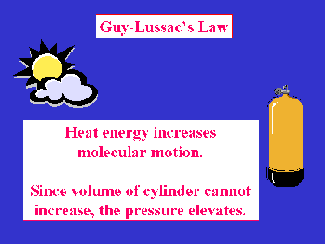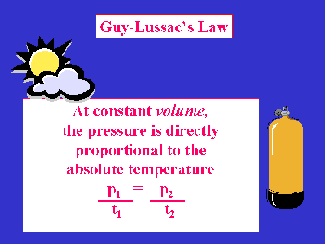ENGLISH EXAMPLE:  A scuba cylinder contains 3000 psig (3014.7 psia) at 78 oF. It is left in the trunk of a car on a hot summer day. If the temperature of the trunk is 115 oF, what will be the gauge pressure of the cylinder?

ANSWER: Using Guy-Lussac's Law (Remember absolute temperature and pressure)

P1   /  T1     =    P2   /  T where

 P = Absolute pressure at conditions 1 and 2 T = Absolute temperature at conditions 1 and 2

First, Determine absolute temperature

T1 =  78 oF + 460  = 538 oR

T2 = 115 oF + 460  = 575 oR

Substituting into Guy-Lussac's Law

3014.7 psia   /   538 oR   =   P2  / 575 oR

Solving:

P2  =  3222 psia

Converting to gauge pressure (gauge reads zero when absolute pressure is one)

3222 psia - 14.7 psi = 3207.3 psig

COMMENT: There is an increase in pressure associated with heating a scuba cylinder. To prevent this increase in pressure (and possible rupture of the safety disc), it is especially important to store full scuba cylinders in a cool place.

METRIC EXAMPLE: A cylinder at 25 oC (298 K) contains gas at a gauge pressure of 200 bar (201.01 bar absolute). Predict the pressure at 42 oC (315 K).

ANSWER: Using Guy-Lussac's Law (Remember Absolute Temperature and Pressure)

Substituting:

201 bar /  298 K   =    P2 /  315 K

Solving:

P2    =   212.5  bar

Converting to gauge pressure:

P2    =   212.5 bar - 1.01 bar

P2    =   211.5 bar

Thus, a scuba cylinder with a gauge pressure of 200 bar at 25 oC heated to 42 oC will show a gauge pressure of about 212 bar.

NOTE: A scuba cylinder is a constant volume device. As kinetic energy increases with temperature, the molecules travel faster. They hit the vessel walls harder and more often. This means pressure, NOT volume, within the cylinder increases as the temperature is raised

BOYLE'S LAW

In 1660, Sir Robert Boyle published the classic "The Spring Of Air And Its Effects" in which he measured the relationship between pressure and volume at constant temperature. He measured the volume of air trapped at the small end of a J-shaped tube. The tube was filled with mercury and the volume of the air space was measured. Adding mercury, (increasing the height of mercury in the J-tube) decreased the volume of air trapped at the small end of the J-shaped tube. He noted that the product of the pressure (as determined by the height of the mercury column) and the volume was constant.  Expressed mathematically:

P V = k       where

 P   = Absolute pressure (height of mercury in tube) V   = Volume (of air space in tube) k   = Constant

This relationship, PV = k, held for a variety of P, V combinations. In mathematics, products equal to the same value can be set equal to each other. This gives us Boyle's Law:

P1 V1 = P2 V2

 P = Absolute pressure at conditions 1 and 2 V = Volume at conditions 1 and 2

Boyles' Law states

The volume of a gas at constant temperature, varies inversely with the pressure.

A corollary to this law states that density (mass/volume) increases directly with the pressure.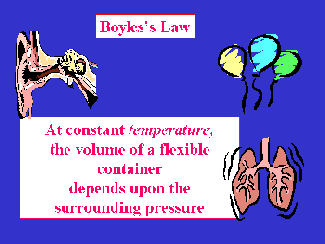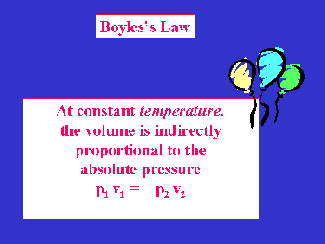ENGLISH EXAMPLE: What is the physical volume (in cubic feet) of an aluminum "80" scuba cylinder?

ANSWER: An aluminum 80 delivers 80 cubic feet of air at one atmosphere (14.7 psia) when filled to a pressure of 3000 psig (3014.7 psia).  Thus, the physical volume of the tank will be the volume at 3000 psig (3014.7 psia)

Substituting into Boyle's Law:

P1 V1 = P2 V2

(14.7 psia) (80 ft3)  =  (3014.7 psia) V2

Solving for V2:

V2  =  0.39 ft3

This physical volume represents how much water the cylinder would hold if the valve were removed and the cylinder were filled with water. This is the value that Europeans refer to as the "water capacity" of a scuba cylinder.

NOTE: Scuba divers carry a small physical volume on their back (0.39 cubic feet, in this case), but have large volumes of available, breathable air (80 cubic feet at sea level) because the air is stored at high pressure and released at ambient pressure by a mechanical device, the regulator.

METRIC EXAMPLE: A scuba cylinder is rated at 2400 l with a pressure of 200 bar. What is the physical volume (water capacity) of the cylinder.

ANSWER: The cylinder delivers 2400 l if all the air is released at 1 bar. The physical volume (water capacity) of the cylinder is the volume of gas compressed to 200 bar. The volume can be found by using Boyle's law:

P1 V1 = P2 V2

Determine absolute pressure: gauge + atmospheric

200 bar + 1 bar = 201 bar

Substituting into Boyle's law

:

P1 V1 = P2 V2

(1 bar) x (2400 l)  = (201 bar)  V2

Solving:

V2  = 11.9 l

ENGLISH EXAMPLE: A scuba cylinder has a rated capacity of 80 ft3 on the surface. Determine the volume of air from this cylinder that will be available to the diver at 33, 66, 99 and 132 fsw.

ANSWER: Boyle's law allows calculation of decreasing volume of air with increasing depth.

Determine absolute pressure: (Hydrostatic pressure + Atmospheric pressure):

For 33 feet

33 fsw  x   33 fsw/atm  =  1 atm

1 atm + 1 atm = 2 ata  (ata = absolute pressure in units of atmospheres)

For 66 feet:

66 fsw  x   33 fsw/atm   =  2 atm

2 atm + 1 atm = 3 ata

For 99 feet:

99 fsw  x  33 fsw/atm    =  3 atm

3 atm + 1 atm = 4 ata

For 132 feet:

132 feet   33 fsw/atm = 4 atm

4 atm  + 1 atm = 5 ata

Substituting into Boyle's Law:

P1 V1 = P2 V2

For 33 fsw:

(1 ata)(80 ft3) = (2 ata) V2

V2 = 40 ft3

For 66 fsw:

(1 ata)(80 ft3) = (3 ata) V2

V2 = 26.7 ft3

For 99 fsw

(1 ata)(80 ft3) = (4 ata) V2

V2 = 20 ft3

For 132 feet:

(1 ata)(80 ft3) = (5 ata) V2

V2 = 16 ft3

The answers are summarized in the following table:The volume shown is the volume calculated for a 80 ft3 cylinder. The fraction represents the proportional amount of the surface volume at that absolute pressure available from ANY size scuba cylinder. The % change represents the difference in volume between each successive 1 ata pressure change.

METRIC EXAMPLE: The corresponding Metric Table for a 2000 liter cylinder: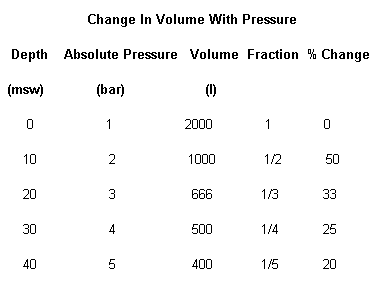The volume shown is the volume calculated for a 2000 liter  cylinder. The fraction represents the proportional amount of the surface volume at that absolute pressure available from ANY size scuba cylinder. The % change represents the difference in volume between each successive 1 bar pressure change.

Remember - as pressure increases, volume decreases. Since breathing is basically a constant volume operation, this is the equivalent of saying that the deeper you go, the less breathing gas will be available to you and thus, the sooner you will consume the air contained in your scuba cylinder. (See  Estimating Gas Consumption ) Likewise, as pressure decreases (as on ascent on scuba), the volume of gas in the lungs and other air spaces will increase. Since the physical size of the body cavity (lungs. ears, sinus, etc.) containing the air is limited, the volume of expanding gas either properly vents through open unobstructed passages or increases by damaging or rupturing tissue. (Analogous to increasing the size of a chewing gum bubble by exhaling into it until it bursts.). As the table clearly shows, the greatest volume change per unit of pressure is in the vicinity of the surface. This means the most risk of a pressure-volume type injury ("Squeezes" and barotraumas) is near the surface.

GENERAL GAS LAW

Any two of the three gas laws of Boyle, Charles or Guy-Lussac can be combined into a relationship termed the General or Combined Gas Law:

P1 V1 )  T1  =  (P2 V2 )  T2

This relationship can be used to predict pressure, volume, and temperature relationships where any five of the six variables are known.

ENGLISH EXAMPLE: The gas in a scuba cylinder occupies a volume of 72 ft3  at 78 oF on the surface. What volume of gas is available to the diver at a depth of 126 ffw and a temperature of 40 oF?

On the surface:

P1  = 1 ata

At 126 ffw

:     P2  =  126 ffw x 1 atm /34 ffw = 3.7 atm

(In fresh water; 34 ffw = 1 atm)

3.7 atm + 1 atm = 4.7 ata

P2 = 4.7 ata

Determine absolute temperature:

T1  =  78 oF + 460 = 538 oR

T2  =  40 oF + 460 = 500 oR

General Gas Law:

( P1 V1 )  T1  =  (P2 V2 )  T2

Substituting:

(1 ata) (72 ft3 )   /   538 oR     =     (4.7 ata) V 500 oP

Solving:

V2 =  14.2  ft3

This demonstrates the decrease in available breathing gas volume associated with descent to deep, cold fresh water that might be encountered in the Great Lakes of the United States.

METRIC EXAMPLE: Calculate the volume of gas available to a diver when a cylinder having a volume of 2000 l with a gauge pressure reading of 200 bar at 25 oC (298K) is used at a depth of 20 msw (3 bar) and a temperature of 30 oC (303K).

ANSWER: Substituting into General Gas Law:

(1 bar) (2000 l)   /    298 K  =   (3 bar) V2   303 K

Solving:

V2  =  678 l

Since it is most likely that both temperature and resultant pressure will vary between the filling of a scuba cylinder and its use, the General Gas Law will give a slightly more realistic evaluation of volume available at depth than a relationship that only examines two of the three pressure- temperature-volume variables.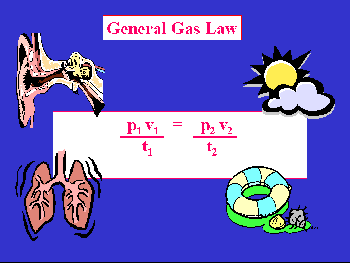DALTON'S LAW

The English chemist John Dalton with collaborator William Henry (of Henry's Law) observed in 1810 the pressures obtained when gases were mixed n the same container. He concluded that when gases were mixed in a container, each gas behaved as if it were the only gas present. Thus, the total pressure in a closed system can be obtained by summing the pressures of each individual component.  The pressure of each individual component is termed the partial pressure.  Expressed mathematically:

p(total) =  p1 + p2 + p3 + .pn

where n   = maximum number of components in the gas mixture

.

The total pressure of a gas mixture is the sum of the partial pressures of all the components.

Since the distances between gas molecules are so vast,  each gas molecule behaves as if it were alone. So, even though components being mixed have pressures of their own, when combined in a container at near atmospheric pressures, the total pressure will be simply the sum of the individual components. However, under high pressure, the volume of gas molecules, compared to the total volume of space available, become significant and this simple summing of pressures no longer applies.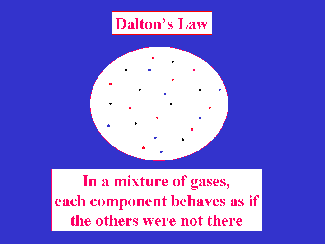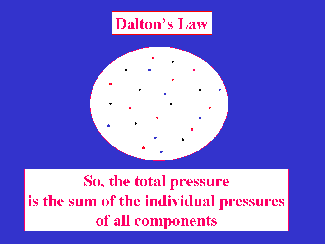Again, this concept is best illustrated with numerical examples.

EXAMPLE:  A one cubic foot (28.3 l) container contains 500 psig (34 bar) of nitrogen gas. Into the container, an additional 346 psig (23.8 bar) of oxygen gas is introduced. Determine the final pressure.

p(total) =  p1 + p2

Substituting:

ENGLISH:

p(total) = 500 psi + 346 psi

METRIC:

p(total) = 34 bar  + 23.8 bar

Solving:

ENGLISH:

p(total) = 846 psi

METRIC:

p(total) = 57.8 bar

Another way of viewing Dalton's Law

pn  =  P(total) x   fraction gas(n) by volume

EXAMPLE: Determine the partial pressure of oxygen in compressed air at a depth of 88 fsw (26.8 msw)

pn  =  P(total) x   fraction gas(n) by volume

Remembering that Air = 21 %  O

Determine absolute pressure

ENGLISH:

P (Hydrostatic) =    88 fsw x  1 atm / 33 fsw  = 2.7 atm

METRIC:

P (Hydrostatic) =   26.8 msw x   1 atm / 10.1 msw  =    2.7 atm

P (absolute)    =  2.7 atm + 1 atm   = 3.7 ata

Substituting:

p O2 = 3.7 ata x 0.21

p O2 = 0.77 ata

EXAMPLE: Determine the partial pressure of nitrogen (78% of air) at the same depth.

p N2 = 3.7 ata x 0.78

p N2 = 2.89 ata

NOTE: The large partial pressure of nitrogen at depth is one of the reasons for the accumulation of nitrogen within the diver. Since nitrogen is physiologically inert (not consumed by metabolism), this nitrogen accumulation can be detrimental to the diver.

HENRY'S LAW

Whenever a gas is in contact with a liquid, gas will dissolve in the liquid. There is a continual movement of gas molecules which are simultaneously moving out of solution into the gas phase and moving from the gas phase into solution within the liquid phase. Although it is impossible to predict the behavior of an individual gas molecule, the net movement of gas will equilibrate such that the partial pressure of the gas going into solution is the same as the partial pressure of the gas coming out of solution. When the gas reaches the state where the amount of gas going into solution is the same as the amount of gas molecules coming out of solution, the solution is said to be saturated with gas. This state is termed equilibrium. At this point, although individual gas molecules will move at random into and out of solution, there will be no net change in gas concentration within the solution.

Henry's Law states

The amount of gas that will dissolve into a solution is directly proportional to the partial pressure of that gas and inversely proportional to the absolute temperature.

The greater the partial pressure of the gas, the greater the driving force for solution and the greater the amount of gas that will dissolve into solution. As the temperature decreases, more gas will dissolve into solution. It is very important to realize that Henry's law is concerned with the amount of gas in solution when equilibrium is reached. It specifically does not address how rapidly that state is reached!

The dissolution of nitrogen within body tissues is approximated by Henry's Law. The deeper one dives, the greater the partial pressure of nitrogen (and any gas in the gas mix) and the greater the gas load each tissue must bear. Upon ascent, the partial pressure in the gas phase decreases. The gas in solution will then escape from solution in an attempt to obtain equilibrium. If this escape from tissue is too rapid for the body to handle, decompression sickness is the result. This is discussed in more detail in the physiology and decompression chapters of this text.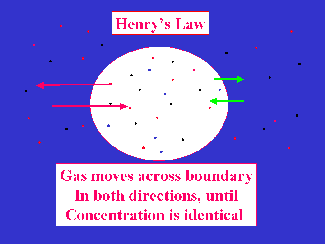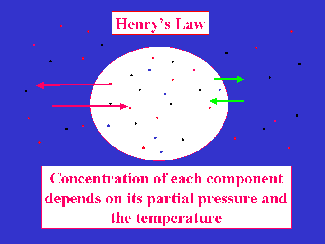An adiabatic system is one in which no heat is added or removed. For an ideal gas (See section on Real vs. Ideal) the following relationship:

Cv  ln ( T2 / T1 )  =  -R ln  (V2 / V1)      where

 Cv = Heat capacity at constant volume ln = Natural logarithm T  = Absolute temperatures at condition one or two R = Universal Gas Constant (see below) V = Volume

Thi    This  equation can be used to calculate the temperature change following an adiabatic change in volume. The mathematics indicates an increase in temperature during compression and a decrease in temperature on expansion of an ideal gas. Thus, the diver notices the scuba cylinder gets hot when filled (The air has been compressed, has less volume, so temperature goes up.) This increase in temperature can be substantial, as much as 1500 oF during rapid compressions. In the presence of hydrocarbon (greases and most lubricants) contaminants, this heat can serve as an energy source for fire and/or explosion in an oxygen enriched atmosphere. When air is rapidly released from a scuba cylinder, either from directly opening the valve to release its contents prior to inspection or by using the purge valve, the volume of the gas increases, so temperature must go down. (The adiabatic cooling associated with gas movement driven by high pressure through a tiny orifice is called the Joule-Thompson effect.) Likewise, as offshore wind moves up a mountain slope, it expands from the decrease in pressure, and cools. Eventually, the cooling will result in the temperature falling below the dew point and clouds or fog then form.

The following discussion on real and ideal gas laws is beyond the scope of recreational diving. The differences between real and ideal gas behavior is, for the most part, insignificant for the diver using compressed air within the traditional recreational diving standards. However, these differences can be substantial when using gas mixtures different from air. Since some within the recreational diving community are beginning to explore the realm of breathing mixes other than air. For that reason, this expanded discussion of real and ideal gas behavior is furnished as a reference.

EXPANDING ON BOYLE: THE REAL GAS LAW

Remember that Boyle measured the product of pressure and volume and always got the same number:

PV  = k

Scientists wanted a single equation that would, without having to measure multiple volumes, temperatures, and pressures give reliable pressure, temperature, volume, AND quantity measurements on gases. This led to an investigation of this constant k. It turns out that temperature and the quantity of a gas can be added to this equation. This new generalized gas equation takes the form:

PV = nRT    where

 P = Absolute pressure V- Volume n = Number of moles present R = Universal Gas Constant (see below) T  = AbsT = Absolute temperatures at condition one or two

A Mole is a chemical measurement of quantity. It is used to relate compounds of different molecular weights. One mole (a mass equal to M grams, where M is the molecular weight of a substance) contains Avagadros' Number (6.024 x 1023) of molecules. For example: one mole of O2 (molecular weight of 32) is 32 grams; this mole of oxygen contains the same number of atoms as 28 grams, one mole, of N2 (molecular weight of 28). Chemists use moles because physical and chemical properties are determined by the numbers of molecules present. Using moles allows chemists to compare substances that have different molecular weights and densities. The mole is also important because it turns out that one mole (the molecular weight (MW) of a gas expressed in grams) at so-called standard temperature and pressure (STP; 0 oC, 1 atm absolute) always has the same volume, 22.414 liters. Thus, using this equation, it is possible to derive not only pressure, temperature, and volume relationships, but quantities of a substance as well.

R is the "universal gas constant" - i.e., it's a number that has been placed into the equation so that the numbers calculated are similar to the numbers observed. (A common trick in science is to add "constants", or fudge factors, to make equations reflect observed reality.) R has been determined from numerous physical measurements. It is equal to the value of PV/nT. (Note: the numeric value of R will vary dependent on the units chosen to measure pressure, volume, and temperature.)

EXAMPLE: How many liters would 5 moles of any gas occupy at 25 oC (298 K) and 2 atm absolute pressure.

PV = nRT; R = 0.082 l-ata K-mole

Substituting:

(2 ata) V = (5 moles) (0.082  l-ata ) (298 K) / K-mole

Solving:

V = 61.1 l

This, 5 moles of gas would correspond to:

5 moles x 32 g / mole  = 160 g  O2    (Oxygen, MW = 32)

5 moles x 28 g / mole  = 140 g  N2   (Nitrogen, MW = 28)

5 moles x   4 g / mole  =  20 g  He     (Helium,  MW =  4)

NOTE: 20 g of He occupies the same volume as 160 g of O2. This is because 20 g of Helium (5 moles) contains the same number of molecules as 160 g (5 moles) of Oxygen. This is why moles, not grams (or any other mass units), are used in chemical equations.

EXAMPLE: Predict the volume of one mole of gas at 5.1 ata and 3 oC (276 K).

PV = nRT

Rearranging:

V = nRT / P

Substituting:

V = (1 mole) (0.082  l-ata)  276 K  /  K-mole

5.1 ata

V =  4.44 l

The following material is beyond the scope of most recreational diving. It does have some relevance in so-called "technical diving" where pressures and gas mixes create situations that are not reliably addressed with the simplistic linear curves of the ideal gases.

REAL & IDEAL

The equation: PV = nRT is called the ideal (or perfect) gas law. It is used to predict the behavior of so-called Ideal Gases. An ideal (or perfect) gas is a gas that exactly behaves according to the laws of Charles and Boyle. In other words, for an ideal gas, the product of PV is always constant (the k in Boyle's law is always equal to nRT) In reality, NO GAS IS IDEAL! This means the product PV does not always remain a constant value. It turns out that most gases, at conditions near STP (standard temperature and pressure; 0 oC, 1 ata) behave according to Boyle's and Charles' Law. As temperature and pressure move away from STP, values calculated by the ideal gas laws, including Boyle's and Charles', are different from the values measured experimentally. The ideal gas situation is best suited to high temperatures and low pressure (when the distances between individual gas molecules is the greatest, so molecular volume is insignificant compared to the container volume).. At scuba cylinder pressures, gases no longer are ideal and as such, ideal equations no longer accurately predict volume and pressure measurements. This deviation from ideal behavior has been explained by the fact that molecules do occupy space. ( An ideal gas molecule occupies zero volume.) Since moving molecules cannot freely move unhindered in all directions, the volume appears larger than predicted by ideal behavior. Also, slight forces of attraction (Van der Waals forces) exist between molecules so that individual molecules truly do not act totally independent of each other. This makes the volume appear smaller than predicted for ideal behavior.

The proximity of molecules to each other will depend on both temperature and pressure. Low pressures and high temperatures keep molecules apart and allow gas behavior to be close to that predicted by the Ideal equations. However, low temperatures and high pressures (like that found in a scuba cylinder) tend to decrease molecular distance and significant difference from Ideal behavior is observed. Such behavior is termed real behavior and equations that predict gas behavior in regions where the simple ideal laws are inadequate are termed real equations.

Under ordinary conditions, the deviation between real and ideal gas behavior is of little concern to divers. However, at scuba cylinder pressures, the difference between real and ideal calculations can be substantial. This difference is particularly important when calculating components for a breathing mixture other than air.

VAN DER WAALS' EQUATION

Since measurement of the pressure and volume of a number of gases at different conditions clearly demonstrated that the simple ideal gas law was inadequate to predict observed behavior, it became necessary to "modify" the ideal gas equation.  Near the end of the 19th, a Dutch chemist, Johanns Van Der Waals, examined the ideal gas equation and made the following assumptions:

1. At low pressures, the intermolecular attractive forces act to cause a decrease in pressure. This causes the product PV in the Ideal gas equation to be lower than expected.

2. At high (scuba-cylinder) pressures, the volume occupied by individual molecules is significant with respect to the total volume occupied by the gas. (At high pressures, the density of the gas is greater. Thus, there will be more molecules per unit volume and the percent volume occupied by gas molecules will increase.) Since the term V in the Ideal gas equation should represent only free space available for gas movement, a correction would be needed to account for the volume of space occupied by gas molecules. Since this correction factor is not present in the Ideal gas law, values calculated for PV at high pressures are larger than measured.

In order to make the ideal gas law more closely conform to observed parameters, Van Der Waals introduced the following modifications:

1. The ideal pressure could be represented as:

P (ideal) = P +  (an2 / V2 )      where

 P = Absolute pressure measured V = Volume a = Constant, characteristic of each gas n = Number of moles present

The constant a represents the attraction between molecules; it is different for each gas and has been determined from empirical observations.

2. The ideal volume could be represented as:

P (ideal) = V -nb    where

 V  = Volume measured b  = Constant, characteristic of each gas n  = Number of moles

The constant b represents the excluded volume of the molecules that make up the gas; it is different for each gas and it has been determined from numerous measurements. Tables of a and b values for various gases are available. One of the most common of such data can be found in the CRC Handbook Of Chemistry And Physics. Values can also be located in almost any chemical engineering textbook. The constants a and b are for pure compounds only and values for mixtures, except air, are commonly not found.

Adding these new P & V terms to the ideal gas law gives rise to the Van Der Waals' equation for real gases. This equation has also been called the real gas law:

(P + an2)/V2 ) (V - nb) = nRT

This equation can be used to derive pressure, temperature, volume, and composition predictions for conditions away from STP. At STP, a/V2 approaches zero and b becomes very small compared to V; thus the Van Der Waals' equation (by substituting 0 for constants a and b) reduces to the Ideal gas equation.

REAL VS. IDEAL CALCULATIONS

The difference between real and ideal is best illustrated by numeric example.

EXAMPLE: Determine the pressure in a scuba cylinder filled with air using both real and ideal gas laws.

ANSWER: Assume a scuba cylinder has a volume of 0.4 cubic feet (from Boyle's Law problem above.) This corresponds to a scuba cylinder volume of:

 0.4 ft3   x   (12 in)3   x (2.54 cm)3  x  1  l        =   11.3 l cylinder         ft3              in3            1000 cm3

Assume this scuba cylinder contains 80 cubic feet (about 2266 l) of gas at atmospheric pressure. Since one mole of gas occupies 22.4 liters at STP, we can approximate the number of moles at STP:

2266   l  /   22.4 l/mole  =  101.2 moles

To simplify our arithmetic, we will assume a scuba cylinder contains about 100 moles of air.

We will also use 25 oC (298 K) as our temperature.

R  is 0.0821 l-ata/ K mole)

IDEAL GAS LAW SOLUTION:

Using the Ideal Equation:

PV = nRT

Rearranging:

P  = nRT / V

Substituting:

P  = (100 moles) (0.0821 l-ata/deg K moles) (298 K)

11.3 l

Solving:

P = 216.5 ata  (This corresponds to 3182.5 psia or 3168 psig)

Now, let's compare this to the pressure predicted from Van Der Waals' real gas equation. The values for a and b were obtained from Table 3.3 in the chemical engineering chapter on gas calculations by Himmelbau cited in the reference section. When working gas law equations with R or other constants, it is important that the values used have consistent units. (For example, it is common for R to be listed in units of l-ata/K-mole. This means pressure must be in ata and volume must be in liters for the obtained solution to be correct. The units for constants a and b in the Van Der Waals' equation must be consistent with the units chosen for R, pressure, and temperature. Tables listing values for R, a, and b with a variety of units are available.)

REAL (VAN DER WAALS' EQUATION) SOLUTION

Using: Van Der Waals' Equation of State:

(P + an2/V2) (V -nb) = nRT

Rearranging:

P =   nRT     -       n2a

(V-nb)             V2

Substituting:

P = (100 mole)(0.0821 L-ata/K moles)(298 K)   -  (100 mole)2  (1.33 L2-atm/mole2)

(11.3 l - 0.036 L/mole (100 mole))                                       (11.3 L)2

P =  317.738 ata - 104.159 ata

P =  213.58 ata (This corresponds to 3139 psia or 3124 psig)

NOTE: There is a difference between the pressure obtained from the real relationship (3124 psig) and the ideal equation (3168 psig) for the pressure exerted by the same physical quantity (100 moles) in the same volume scuba cylinder. For most recreational scuba diving, this difference (about 1.4% for air) is insignificant. However, when pressures of gases are used to determine the composition of breathing gases other than air, then the difference between the real gas composition and the gas composition predicted from the simpler, ideal gas behavior can be life threatening. This difference is particularly true for helium containing mixtures.

COMPRESSIBILITY

Another approach to resolving the dilemma between ideal and real behavior is the concept of compressibility. In this scheme, the formula for predicting gas behavior is

PV = znRT   where

z = compressibility factor based on empirical observations

Once again, science introduces a constant (an empirically derived measurement) to make an equation fit reality. The value z is different for each gas and varies with the pressure and temperature. Tables and graphs to find the appropriate "z-factor" at needed conditions are available. Note that for an ideal gas, z = 1 and the real "compressibility" equation reduces to the ideal gas law.

BEYOND REAL

The Ideal gas equations adequately predict gas behavior at conditions near STP. As conditions move away from STP, more terms have to be added to the equations so that the predictions are close to observed values of pressure, temperature, volume, and composition. These new terms gave rise to the real gas law. The real gas equation of Van Der Waals provides enough correlation with observed values to be used at scuba cylinder pressures. However, as the pressure continues to increase, the so-called real gas law begins to deviate from observed values and additional terms must be added to this real equation in order for calculated values to correlate with observed gas behavior. These equations are well beyond the scope of recreational diving.

CONCLUSION

Lou Fead, the "Easy Diver," said it best with "Dive with your brains, not your back!" Understanding the physical principles that govern the environment in which you have chosen to play will enhance both your enjoyment and your safety as you emerge yourself in Planet Ocean.

Ames, JS, The Free Expansion Of Gases: Memoirs of Gay-Lussac, Joule and Joule and Thompson, Harper and Brothers Publishers, New York, 1898

Barus, C Harper's Scientific Memoirs: The Laws of Gases, Harper & Brothers Publishers, New York, 1899

Blake. W & Mossman, Matheson Gas Data Book, Matheson Gas Products, Milwaukee, 1971, p 273

Boyle, R. New Experiments Phyiomechanical Touching The Spring Of The Air And Its Effects, a chapter in  Runes D (Ed) Treasury of World Science, Littlefield Adams& Co, Patterson, NJ. 1962, 73-94.

Calhoun, F Physics For Divers, NAUI, Colton, 1978

Carlucci, P Pletzke, T. & Peuglar, R. Gas Mixtures: Facts & Fables, Matheson Gas Products, Secaucus,1991

Dickens, R The Physics And Engineering Of Diving, D.L.S. Enterprises, Jacksonville,1981

Hammelblau, DM Basic Principles and Calculations in Chemical Engineering, Prentice-Hall, 1982, p. 224

Parbrook, G Davis, P & Parbrook, E Basic Physics And Measurement In Anesthesia, Butterworth-Heinemann Ltd, Boston, 1990

Scornavacca, F Gas-Mixtures: Facts & Fables, Matheson Gas Products, Secaucus, 1975

Snider, E (Ed.) V1: Introduction & Computations For Gases, 1980:  V4:Ideal Gas Law, Enthalpy, Heat Capacity, Heats Of Solution And Mixing, American Institute Of Chemical Engineers, New York, 1984

Tucker, D & Gazey, B Applied Underwater Acoustics, Pergamon, New York, 1977

Weast, RC Handbook of Chemistry and Physics, CRC Press, Boca Rotan, 1973a, pp. F-11, B-16

Weast, RC Handbook of Chemistry and Physics, CRC Press, Boca Rotan, 1973b, D-157

Webb, .P Thermal Problems, A chapter in Bennett, P & Elliot, D The Physiology and Medicine of Diving, Best Publishing, San Pedro, 1982, p. 302

Wienke, B Basic Diving Physics And Applications, Best Publishing, Flagstaff, 1994

Wienke, B Basic Technical Diving In Depth, Best Publishing, Flagstaff, 2001

Top

Jump To: Kinetic     Absolute Values     Charles     Guy-Lussac     Boyle     General     Dalton     Henry     Real     VanDer Waal

Fini

Credit:

Portions of this article  were used in my chapter on Dive Physics appearing in:

Bove and Davis' Diving Medicine (4 th Edition), published by Saunders (Elsevier)

Larry "Harris" Taylor, Ph.D. is a biochemist and Diving Safety Coordinator at the University of Michigan. He has authored more than 100 scuba related articles. His personal dive library (See Alert Diver, Mar/Apr, 1997, p. 54) is considered one of the best recreational sources of information In North America.

Copyright 2001-2023 by Larry "Harris" Taylor